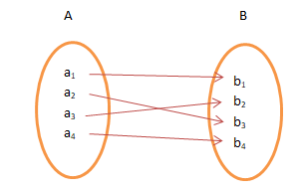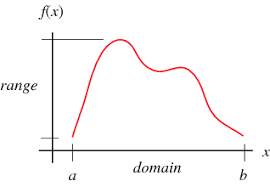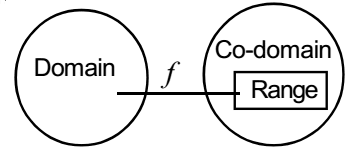Learn US

# Codomain, Domain and Range

811 views

 1 Introduction 2 Domain and Range 3 Codomain 4 Range vs Domain 5 Codomain vs Range 6 Domain vs Codomain 7 Summary 8 FAQs

25th September 2020

## Introduction

Some of the most important aspects of a function include Domain, Range, and Codomain. These three basic concepts are the foundation for defining any function. In fact, these may as well make or break an entire function. Hence it is important to have knowledge about each. While domain and range are very commonly talked about concepts, Codomain is the lesser spoken about but still very well an important part of a function which we will explain in this blog. So we will start by defining all three.But most importantly, we will cover the differences between the three terms, codomain vs range, range vs codomain, domain vs codomain

## Domain and Range

Domain is defined as the set of all the values that the function can input while it can be defined. Range, are all the values that come out as output of the function involved.

Example

f(x) = x2

If the domain is taken as the set of all integers while the range is coming out to be only a positive set of numbers which are squares i.e. 0, 1, 4, 9…..

if , Domain = Z then

Range = {0, 1, 4, ….}

## Codomain

This is the set of values that have the potential of coming out as outputs of a function.

Example

y = x2

Since it is a function defined from R->R, all real values are potential values that can come out. Hence, the codomain could be the set R.

## Range vs Domain

Perhaps the easiest to grasp, let us look at the difference between domain and range.

As mentioned earlier, the domain is the set of input values that are defined on a function. While the range is all the output values, hence there lies the first basic difference between domain and range.

While domain associates with the input of the function, range associates purely with the actual output.

Now taking in terms of the variables involved, let us take a function f(x). Domain will pertain to all possible values of x, which is the independent variable. Range on the other hand will pertain to the entirety of f(x) which is the dependent variable. There lies the second difference between  domain and range.

One major difference also lies with the graphical representation. Since we established that  domain is the x values, and range is all the f(x) values, the domain will be represented in the x=-axis while the range in shown in the y-axis.Also, it should be noted that range deepens entirely on the values of domain.

Let us take an example,

Let us take the movement of the two arms of a clock. So while the time is the domain. The angle between the two hands is a dependent variable at the time of the clock. Hence that would be the range.## Codomain vs Range

One of the biggest confusions in these concepts is what is Codomain VS Range. But there are some very fundamental differences between codomain and range which aren’t that obvious like say in range vs domain.

Let us carefully observe the two definitions.

The range is the set of all values which are actually coming out as output because of certain values being input into the function. So these are all the values that have to have a certain value of the input necessarily which means for all the values of the range being equal to f(x) there is necessarily a value of x that exists.

On the other hand, codomain is the set of possible outcomes. So they have a potential to be f(x) but it is not necessary that the values existing in codomain will come out as outputs or will have a certain value in the domain for them.Also, since codomain values might not actually be present as outputs, they cannot necessarily be put on the y-axis against a certain x-value. Thus, its graphical representation isn’t possible for a function.
But there is a possibility that range is equal to codomain, then there are special functions that have this property and we will explore that in another blog on onto functions. Then if range becomes equal to codomain the n set of values wise there is no difference between codomain and range.

Example

Taking an example of the clock and the angles between the hands, the codomain of such a function could be all angles. But since the hands only move around a 360 degrees circle, we won’t have angles more than that. And thus the codomain could include angles like 900, 1400, etc, but we will have a range of values that will be restricted.## Domain vs Codomain

The third and last difference is Domain Vs Codomain. While this is similar to Range vs Domain in the sense that domain deals with input values and the x in f(x), codomain deals with potential f(X) outputs.

Also, again domain deals with the independent variable while codomain gives us a loose idea about the dependent variable.

But where this difference varies from Range vs Domain is that graphically codomain can be represented separately but not in the graph of the function. Hence, the actual f(x) graph will have domain necessarily on the x-axis but not the codomain since many of the values of codomain could not even be there in f(x).Also, the codomain doesn’t depend on domain values as the range does.

## Summary

This blog covered domain and range, codomain, and the various differences range vs codomain, domain vs codomain, and range vs domain. On closer inspection of the definitions of all three, the difference between domain and range came out to be very clear and similar to the difference between domain and codomain except for the fact that range deals with actual output values and codomain deal with potential values. This is also what made the difference between codomain and range also very clear. The differences are all with respect to definition, nature of values of the set, kind of variable associated, and graphical representation.

## Difference between domain and range

• While domain associates with the input of the function, range associates purely with the actual output. F(x): Domain will pertain to all possible values of x, which is the independent variable. Range on the other hand will pertain to the entirety of f(x) which is the dependent variable.
• The domain will be represented in the x=-axis while the range in shown in the y-axis.
• Also, it should be noted that range deepens entirely on the values of domain.

## Codomain vs Range

• The range is the set of all values which are actually coming out as output because of certain values being input into the function. On the other hand, codomain is the set of possible outcomes.
• Codomain’s graphical representation isn’t possible for a function.
• If range becomes equal to codomain then set of values wise there is no difference between codomain and range.

## Domain vs Codomain

• Domain deals with the independent variable while codomain gives us a loose idea about the dependent variable.
• The actual f(x) graph will have domain necessarily on the x-axis but not the codomain since many of the values of codomain could not even be there in f(x).
• Also, the codomain doesn’t depend on domain values as the range does.

GIVE YOUR CHILD THE CUEMATH EDGE
Access Personalised Math learning through interactive worksheets, gamified concepts and grade-wise courses